﻿

### Flow-invariant sets

#### Abstract

Let S be a subset of some normed space E and let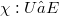$\chi : U → E$ be a continuous function defined on an open subset U containing S. We consider functions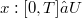$x : [0,T] → U$ which satisfy the differential equation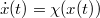$\dot{x}(t)=\chi(x(t))$ for all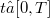$t ∈ [0,T]$, where T is a positive number, depending on z. Such functions will be called solutions of (1). We say that S is invarianC with reqhzt fo (l), if every solution of (1) with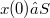$x(0) ∈ S$ remainsin S,that is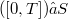$([0,T]) ⊆ S$. Early results conceming the characterization of invariant sets in the finite dimensional case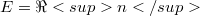$E = \Ren$ were obtained by Nagumo [Na42]. Further research was independently done by Bony [Bo69], Brezis [BGO], Crandall [Cr72], Hartman [Ha721 and Yorke Tyo673, [Yo70]. Different conditions imposed on S and x by Bony and Brezis respectively were related with one another by Redheffer [Re72]. He elucidated as well as generalized their results. There was even some progress in the case of infinite dimensional spaces. Redheffer and Walter [ReWa75] were the first to investigate this field. Their results were extended by Volkmann [Vo73], [Vo75]. Finally, Martin [Ma73] and Volkmann [Vo76] came to farreaching generalizations in case E is a Banach-space. This note offers an alternative approach based on an elementary lemma from the theory of functions of one real variable. As a corollary we obtain a slightly more general version of Brezis’ Invariance Theorem. In this fashion it becomes evident that the latter is a higher dimensional generalization of the lemma from one-dimensional calculus.

DOI Code: 10.1285/i15900932v9n2p221

Full Text: PDF